# Linear regression from scratch

For this article the prerequisite is: Andrew Ng’s linear regression lecture.

clickhere to check out the course.

# Problem Statement:

• Given the Years of experience we have to predict salary of the person. Since dependent variable salary is continuous, it is a regression problem.
• We will use three methods i.e. Gradient descent (Optimization method), Statistical method (formula) and Scikit learn Linear regression library to estimate the regression parameter.
• After that we will compare the parameters learned from all these three methods.

# Importing libraries and Loading dataset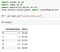Importing libraries and Loading dataset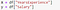X and y are independent and dependent variable respectively

## Plotting X, y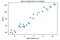Scatter plot to check liner relationship exist
• The graph shows there is a linear positive relationship between Years of experience and salary, which means as Experience increases salary also increases linearly.

## Normalizing the data to make learning fasterMin-Max Scaling
• Min-Max normalization is a scaling technique, for every feature it will bring value between 0 and 1.
• Minimum value will get transformed to 0.
• Maximum Value will get transformed to 1.
• Rest of the value will be between 0 and 1.

Reason: why scaling?

• The one of the important reasons we apply scaling is so that optimization algorithms such as gradient descent will converge faster (will reach to minima faster).

Problem with scaling

• Problem with scaling is it makes parameters hard to interpret.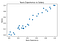Scatter plot after Min-Max scaling
• Scatter plot shows, if we draw a straight line from Years of experience we can predict the salary of the person.
• The equation of straight line is given by: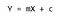Equation of straight line
• We know the value of `X` i.e. years of experience and we also know the value of `y` i.e. salary. We don't know `m` i.e. slope of the line and `c` i.e. y intercept.
• If we would have known `m` and `c` with respect to `X` (years of experience) and `y`(salary) we would have drawn the best fitted straight line.

## What ML algorithm learns.

• In Machine learning terms `m` and `c` are called as the `parameters`, and that is what the machine learns.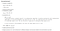How machine learns?

Initialize

parameter (theta_0, theta_1): only when we start training.

itr: list of value of iteration.

cost_itr: list which contains cost at every iteration.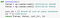Initialize parameters, cost_itr and itr

Predict

Predicting `y` after updating parameter (theta_0, theta_1)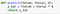predict y

Plotting

It is not required function to implement gradient descent, however it can be used to understand how machine is actually learning.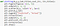It will plot the y_predict line and cost at given iteration, It is not required but it is important to understand the concepts.

Learn

Every important function, It will update parameters at every iteration, It will also call above functions initialize, predict, plotting and help model train and visualize.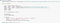Where learning happensStart learning (training) model for 3001 times at 0.009 learning rate

Iteration 0:

Plotting after randomly initialize theta_0 and theta_1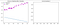Predicting y after randomly initializing theta0, theta1

Iteration 300:

Plotting after some learning happens theta_0 and theta_1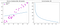Predicting y after 300 iteration and cost vs iteration graph

Iteration 600:

Compare lines at iteration = 300 and iteration = 600, model is improving.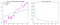Predicting y after 600 iteration and cost vs iteration graph

Iteration 900:

More improved model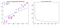Predicting y after 900 iteration and cost vs iteration graph

Iteration 3000:

Finally model is trained for 3000 iteration.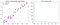Predicting y after 3000 iteration and cost vs iteration graph

# Statistical Approach

Other than gradient descent approach there is statistical approach to find the parameter (m,c) of regression line. The statistical approach is direct or formula based approach to find parameters.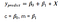Parameters are denoted as beta in statistics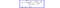Statistical formula for estimating parameters

## Comparing: Actual vs Predicted by Statistical parameter vs Gradient descent parameters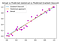Actual vs Predicted by Statistical parameter vs Gradient descent parameters

Statistical approach predicts `y `more accurately than gradient descent approach hence parameters of Statistical approach is more accurate than Gradient descent approach.

## Scikit Learn: Linear Regression API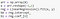Training Linear Regression model using scikit learn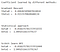Comparing parameters of all three methods

Coefficient estimated by Statistical approach and Scikit learn same, hence the error will be less however there is still improvement in self defined Gradient descent approach.

## Improving Gradient Descent

Training model for 6001 iteration, earlier it was only trained for 3001 iteration.Training Gradient descent for 6001 iteration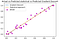Almost got

## Comparing: Actual vs Predicted by Statistical parameter vs Improved Gradient descent parameters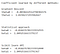Comparing parameters of all three methods

Our improved gradient descent has almost reached near to the best parameter. If we train for few more iterations it will surely find best parameter.

clickhere to check the code

Thank you